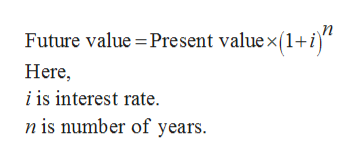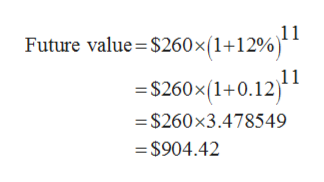# What is the value in year 15 of a $260 cash flow made in year 4 if interest rates are 12 percent? (Do not round intermediate calculations. Round your answer to 2 decimal places.) Question Asked Oct 3, 2019 5 views What is the value in year 15 of a$260 cash flow made in year 4 if interest rates are 12 percent? (Do not round intermediate calculations. Round your answer to 2 decimal places.)

check_circle

Step 1

Future value can be defined as the monetary value of an asset on an individual date in future assuming a specified growth rate.

It is given that,

The amount of cash flow is $26. Growth rate is 12%. Since the cash flow occur in year 4, the interest rate will be applicable for 11 years. Step 2 The formula to calculate future value is given below:help_outlineImage Transcriptioncloseп Future value Present value x (1+i Here i is interest rate n is number of years fullscreen Step 3 Substitute$260 for present value, 11 for n and ...help_outlineImage TranscriptioncloseFuture value $260x (1+12%) 11 S260x(1+0.12) -$260 x3.478549 =\$904.42 fullscreen

### Want to see the full answer?

See Solution

#### Want to see this answer and more?

Solutions are written by subject experts who are available 24/7. Questions are typically answered within 1 hour.*

See Solution
*Response times may vary by subject and question.
Tagged in